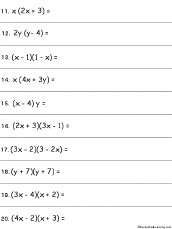More on Math Polynomials Quadratic Equations

A polynomial is a sum or difference of terms; each term is:
• a constant (like 5)
• a constant times a variable (like 3x)
• a constant times the variable to a positive integer power (like 2x2)
• a constant times the product of variables to positive integer powers (like 2x3y).
A monomial is a polynomial with only one term. A binomial is a polynomial that has two terms. A trinomial is a polynomial with three terms.

Degree of a Polynomial: The degree of a term within a polynomial is the sum of the exponents of variables that occur in that term (if there is no exponent written on a variable, such as in 3x, the exponent is one). The degree of a polynomial is the greatest degree of any term in the polynomial (for instance, for the polynomial 4x2 + 7xyz, the degree is 3 because of the last term).

• If a polynomial isn't just a constant and if each term has at most a variable to the first power (like 4x - 2 or 3y), then it is a first-degree polynomial (also called a linear polynomial).
• If a polynomial's highest degree is two, it is a quadratic (or second-degree) polynomial (example: 4x2 + 3x + 7).
• If a polynomial has more than one variable, then its degree is the sum of the exponents of the highest-degree term. For example, the polynomial 2xy2 -3xy + 6x - 2 has degree 3 (the sum of the xy2 exponents, 1 + 2).
Polynomials are often written in descending order, in which the terms with the largest powers are written first (like 9x2 - 3x + 6). If they are written with the smallest terms appearing first, this is ascending order (like 6 - 3x + 9x2).A polynomial equation is an equation involving a polynomial.

Multiplying Polynomials:
Multiply Polynomials: Introduction to Algebra - Worksheets to PrintMultiply Polynomials Worksheet #1Multiply 10 polynomials and put the answers in simplest form. Or go to the answers.Multiply Polynomials Worksheet #2Multiply 10 polynomials and put the answers in simplest form. Or go to the answers.

 Quadratic Equation: ax2 + bx - c = 0Printable Worksheets
MiscellaneousPascal's Triangle - WorksheetFill in the missing entries in Pascal's Triangle. Go to the answers.

Enchanted Learning®
Over 35,000 Web Pages
Sample Pages for Prospective Subscribers, or click below

 Overview of Site What's New Enchanted Learning Home Monthly Activity Calendar Books to Print Site Index K-3 Crafts K-3 Themes Little ExplorersPicture dictionary PreK/K Activities Rebus Rhymes Stories Writing Cloze Activities Essay Topics Newspaper Writing Activities Parts of Speech Fiction The Test of Time Biology Animal Printouts Biology Label Printouts Biomes Birds Butterflies Dinosaurs Food Chain Human Anatomy Mammals Plants Rainforests Sharks Whales Physical Sciences: K-12 Astronomy The Earth Geology Hurricanes Landforms Oceans Tsunami Volcano Languages Dutch French German Italian Japanese (Romaji) Portuguese Spanish Swedish Geography/History Explorers Flags Geography Inventors US History Other Topics Art and Artists Calendars College Finder Crafts Graphic Organizers Label Me! Printouts Math Music Word Wheels

## Enchanted Learning Search

 Search the Enchanted Learning website for: